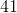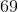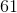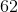# Common Core: 1st Grade Math : Understanding Place Value

## Example Questions

### Example Question #31 : Understanding Place Value

What digit is in the ones place?Explanation:

The ones place is always the first number on the right.

### Example Question #32 : Understanding Place Value

What digit is in the ones place?Explanation:

The ones place is always the first number on the right.

### Example Question #57 : Number & Operations In Base Ten

What digit is in the ones place?Explanation:

The ones place is always the first number on the right.

### Example Question #58 : Number & Operations In Base Ten

What digit is in the ones place?Explanation:

The ones place is always the first number on the right.

### Example Question #33 : Understanding Place Value

What digit is in the ones place?Explanation:

The ones place is always the first number on the right.

### Example Question #60 : Number & Operations In Base Ten

What digit is in the ones place?Explanation:

The ones place is always the first number on the right.

### Example Question #34 : Understanding Place Value

What digit is in the tens place?Explanation:

The tens place is always the second number from the right.

### Example Question #35 : Understanding Place Value

What digit is in the tens place?Explanation:

The tens place is always the second number from the right.

### Example Question #31 : Understanding Place Value

What digit is in the tens place?Explanation:

The tens place is always the second number from the right.

### Example Question #64 : Number & Operations In Base Ten

What digit is in the tens place?